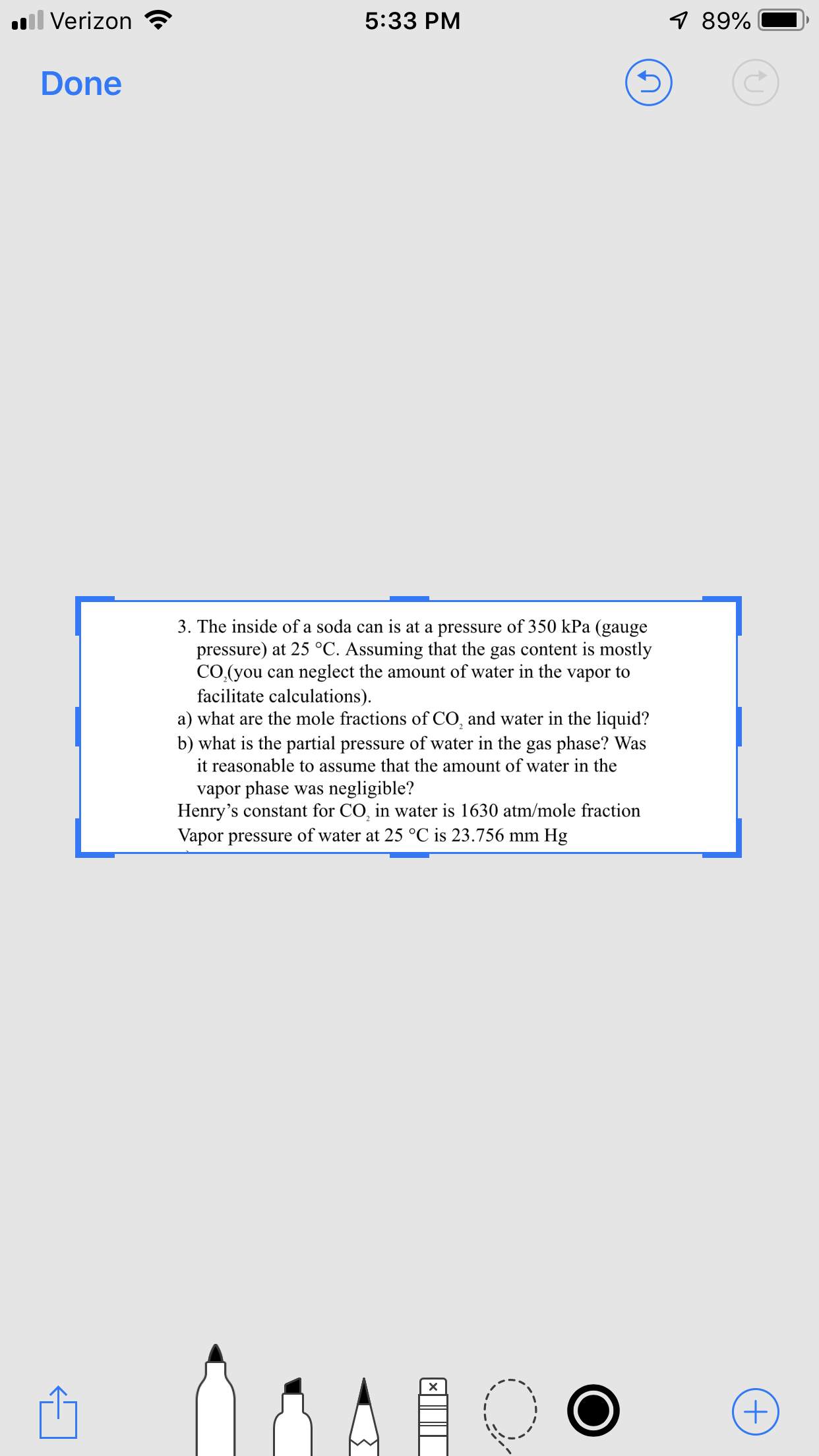# .Verizon89%5:33 PMDone3. The inside of a soda can is at a pressure of 350 kPa (gaugepressure) at 25 °C. Assuming that the gas content is mostlyCO.(you can neglect the amount of water in the vapor tofacilitate calculations)a) what are the mole fractions of CO, and water in the liquid?b) what is the partial pressure of water in the gas phase? Wasit reasonable to assume that the amount of water in thevapor phase was negligible?Henry's constant for CO, in water is 1630 atm/mole fractionVapor pressure of water at 25 °C is 23.756 mm Hg

Question
9 viewshelp_outlineImage Transcriptionclose.Verizon 89% 5:33 PM Done 3. The inside of a soda can is at a pressure of 350 kPa (gauge pressure) at 25 °C. Assuming that the gas content is mostly CO.(you can neglect the amount of water in the vapor to facilitate calculations) a) what are the mole fractions of CO, and water in the liquid? b) what is the partial pressure of water in the gas phase? Was it reasonable to assume that the amount of water in the vapor phase was negligible? Henry's constant for CO, in water is 1630 atm/mole fraction Vapor pressure of water at 25 °C is 23.756 mm Hg fullscreen
check_circle

star
star
star
star
star
1 Rating
Step 1

Part (a)

First convert the given gauge pressure in absolute pressure in atm as:

Step 2

Since the mole fraction of water in the vapor phase is assumed to be negligible, use the formula for henry’s law to calculate the mole fraction of CO2 and H2O  in the liquid phase as:

Step 3

Part (b)

The vapor pressure of water at 25⸰C is given as 23.756 mmHg. The pa...

### Want to see the full answer?

See Solution

#### Want to see this answer and more?

Solutions are written by subject experts who are available 24/7. Questions are typically answered within 1 hour.*

See Solution
*Response times may vary by subject and question.
Tagged in